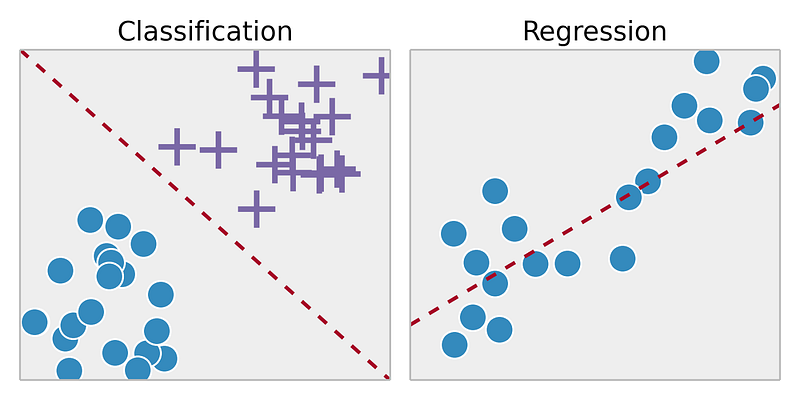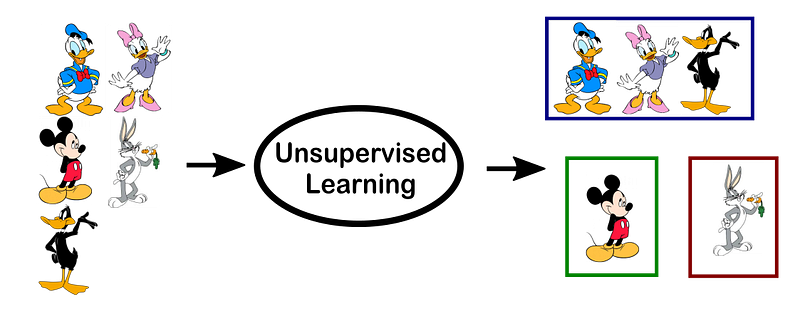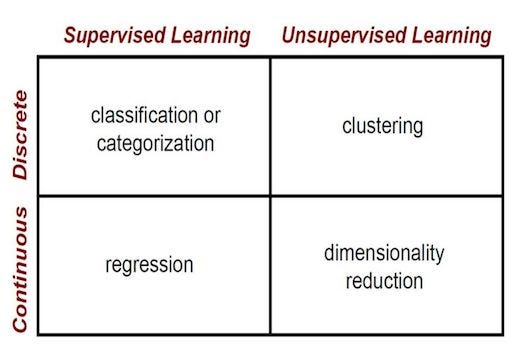Topics: AI | Data Science | Data Visualization | Deep Learning | Machine Learning | NLP | Python | R | Statistics

KDnuggets Home » News » 2018 » Apr » Tutorials, Overviews » Supervised vs. Unsupervised Learning ( 18:n15 )

#Supervised vs. Unsupervised Learning

Understanding the differences between the two main types of machine learning methods.comments

By Devin Soni, Computer Science Student

Within the field of machine learning, there are two main types of tasks: supervised, and unsupervised. The main difference between the two types is that supervised learning is done using a ground truth, or in other words, we have prior knowledge of what the output values for our samples should be. Therefore, the goal of supervised learning is to learn a function that, given a sample of data and desired outputs, best approximates the relationship between input and output observable in the data. Unsupervised learning, on the other hand, does not have labeled outputs, so its goal is to infer the natural structure present within a set of data points.

Supervised LearningSupervised learning is typically done in the context of classification, when we want to map input to output labels, or regression, when we want to map input to a continuous output. Common algorithms in supervised learning include logistic regression, naive bayes, support vector machines, artificial neural networks, and random forests. In both regression and classification, the goal is to find specific relationships or structure in the input data that allow us to effectively produce correct output data. Note that “correct” output is determined entirely from the training data, so while we do have a ground truth that our model will assume is true, it is not to say that data labels are always correct in real-world situations. Noisy, or incorrect, data labels will clearly reduce the effectiveness of your model.
When conducting supervised learning, the main considerations are model complexity, and the bias-variance tradeoff. Note that both of these are interrelated.

Model complexity refers to the complexity of the function you are attempting to learn — similar to the degree of a polynomial. The proper level of model complexity is generally determined by the nature of your training data. If you have a small amount of data, or if your data is not uniformly spread throughout different possible scenarios, you should opt for a low-complexity model. This is because a high-complexity model will overfit if used on a small number of data points. Overfitting refers to learning a function that fits your training data very well, but does not generalize to other data points — in other words, you are strictly learning to produce your training data without learning the actual trend or structure in the data that leads to this output. Imagine trying to fit a curve between 2 points. In theory, you can use a function of any degree, but in practice, you would parsimoniously add complexity, and go with a linear function.

The bias-variance tradeoff also relates to model generalization. In any model, there is a balance between bias, which is the constant error term, and variance, which is the amount by which the error may vary between different training sets. So, high bias and low variance would be a model that is consistently wrong 20% of the time, whereas a low bias and high variance model would be a model that can be wrong anywhere from 5%-50% of the time, depending on the data used to train it. Note that bias and variance typically move in opposite directions of each other; increasing bias will usually lead to lower variance, and vice versa. When making your model, your specific problem and the nature of your data should allow you to make an informed decision on where to fall on the bias-variance spectrum. Generally, increasing bias (and decreasing variance) results in models with relatively guaranteed baseline levels of performance, which may be critical in certain tasks. Additionally, in order to produce models that generalize well, the variance of your model should scale with the size and complexity of your training data — small, simple data-sets should usually be learned with low-variance models, and large, complex data-sets will often require higher-variance models to fully learn the structure of the data.

Unsupervised LearningThe most common tasks within unsupervised learning are clustering, representation learning, and density estimation. In all of these cases, we wish to learn the inherent structure of our data without using explicitly-provided labels. Some common algorithms include k-means clustering, principal component analysis, and autoencoders. Since no labels are provided, there is no specific way to compare model performance in most unsupervised learning methods.

Two common use-cases for unsupervised learning are exploratory analysis and dimensionality reduction.

Unsupervised learning is very useful in exploratory analysis because it can automatically identify structure in data. For example, if an analyst were trying to segment consumers, unsupervised clustering methods would be a great starting point for their analysis. In situations where it is either impossible or impractical for a human to propose trends in the data, unsupervised learning can provide initial insights that can then be used to test individual hypotheses.

Dimensionality reduction, which refers to the methods used to represent data using less columns or features, can be accomplished through unsupervised methods. In representation learning, we wish to learn relationships between individual features, allowing us to represent our data using the latent features that interrelate our initial features. This sparse latent structure is often represented using far fewer features than we started with, so it can make further data processing much less intensive, and can eliminate redundant features.

TLDR:Bio: Devin Soni is a computer science student interested in machine learning and data science. He will be a software engineering intern at Airbnb in 2018. He can be reached via LinkedIn.

Original. Reposted with permission.

Related:

Top Stories Past 30 Days
Most SharedGet KDnuggets, a leading newsletter on AI, Data Science, and Machine Learning